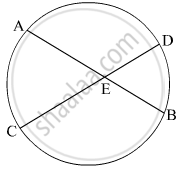Advertisement Remove all ads

# Four Alternative Answers for the Following Question is Given. Choose the Correct Alternative.Chords Ab and Cd of a Circle Intersect Inside the Circle at Point E. If Ae = 5.6, Eb = 10, Ce = 8, Find Ed. - Geometry

MCQ

Four alternative answers for the following question is given. Choose the correct alternative.
Chords AB and CD of a circle intersect inside the circle at point E. If AE = 5.6, EB = 10, CE = 8, find ED.

#### Options

• 7

• 8

• 11.2

• 9

Advertisement Remove all ads

#### SolutionIf two chords of a circle intersect each other in the interior of the circle, then the product of the lengths of the two tangents of one chord is equal to the product of the lengths of the two segments of the other chord.
∴ AE × EB = CE × ED
⇒ 5.6 × 10 = 8 × ED
⇒ ED = $\frac{56}{8}$  = 7 units
Hence, the correct answer is 7.

Concept: Touching Circles
Is there an error in this question or solution?
Advertisement Remove all ads

#### APPEARS IN

Advertisement Remove all ads
Advertisement Remove all ads
Share
Notifications

View all notifications

Forgot password?